## Definition Of Mixed Number

A number that is the sum of a whole number and a proper fraction is called a mixed number.

In other words, a mixed number is a number that has a whole number part and a fractional part.

### Examples of Mixed Number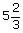is a mixed number where 5 is a whole number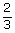is a proper fraction.
The following are few more examples of mixed numbers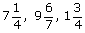.

### Solved Example on Mixed Number

#### Ques: Joe is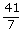ft tall. Write the fraction as a mixed number.

##### Choices:

A.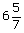B.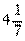C.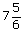D.Step 1:. [Original fraction.]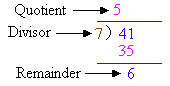Step 3:[Write in the form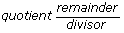.]
Step 4: The mixed number for the improper fractionis.